Courses

# Test: Shapes and Patterns - 2

## 10 Questions MCQ Test Mathematics for Class 2 | Test: Shapes and Patterns - 2

Description
This mock test of Test: Shapes and Patterns - 2 for Class 2 helps you for every Class 2 entrance exam. This contains 10 Multiple Choice Questions for Class 2 Test: Shapes and Patterns - 2 (mcq) to study with solutions a complete question bank. The solved questions answers in this Test: Shapes and Patterns - 2 quiz give you a good mix of easy questions and tough questions. Class 2 students definitely take this Test: Shapes and Patterns - 2 exercise for a better result in the exam. You can find other Test: Shapes and Patterns - 2 extra questions, long questions & short questions for Class 2 on EduRev as well by searching above.
QUESTION: 1

### What is the next figure in the given pattern?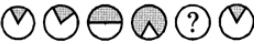Solution:

In each step a little more area of the circle is shaded. So, the next figure must be a fully shaded circle.

QUESTION: 2

### How many more flat faces does solid A have than Solid B?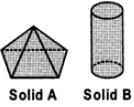Solution:

No. of flat faces in Solid A = 5 No. of flat faces in Solid B = 2
∴ Their difference = 5 - 2 = 3

QUESTION: 3

### Study the given figure and answer the questions that follow.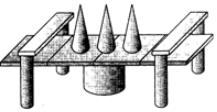Identify the number of cylinders.

Solution:

There are 5 cylinders in the given figure.

QUESTION: 4

Study the given figure and answer the questions that follow.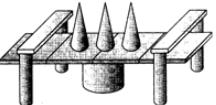How many cuboids are there?

Solution:

There are 12 cuboids in the figure.

QUESTION: 5

Study the given figure and answer the questions that follow.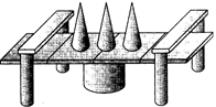How many cones are there?

Solution:

There are 3 cones in the figure.

QUESTION: 6

Study the given figure and answer the questions that follow.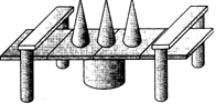How many more cylinders are there than cones?

Solution:

There are 3 cones and 5 cylinders in the figure. So, there are 5 - 3 = 2 more cylinders than cones.

QUESTION: 7

Study the given figure and answer the questions that follow.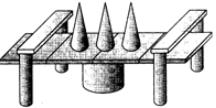How many shapes in all have curved surfaces?

Solution:

3 cones and 5 cylinders i.e., 8 shapes have curved surfaces.

QUESTION: 8

How many squares are there in the. Given figure?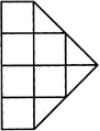Solution:
QUESTION: 9

Find the number of triangles in the figure given.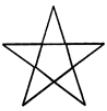Solution:
QUESTION: 10

Find the number of sides in the figure given.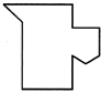Solution: